# Truncate shapes

Â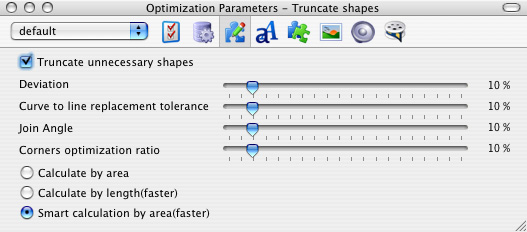Truncate shapes set optimizes vector shapes by removing and replacing groups of shapes with optimized ones

Each physical shape consists of a set of curves which define shape’s outline. By reducing several curves to single one, which is most fit, one may reduce the number of curves, and, in a turn, file size. Most fit curve is a curve, which has minimal ratio between resulting shape’s area and original one.

Flash Optimizer uses the following algorithms:

• Average distance between curves (calculate by length)

Selects several points at the original curve and the new one, connects them and calculate average distance. Now, most fit curve is one that has minimal average distance.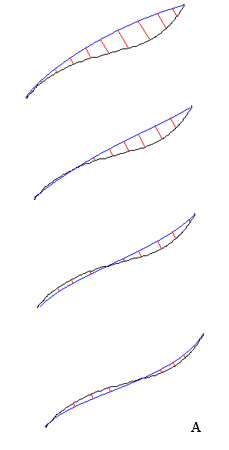Â Â  Â  Â  Â  Â  Â  Â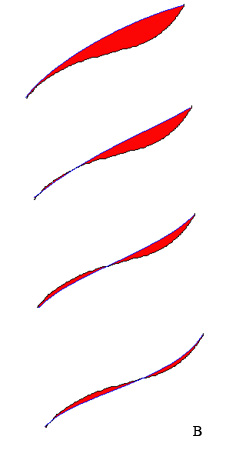At the image A above you may see original curve (black), and new curve (blue). They are connected with a set of lines (red). Now, we calculate average length of these lines. Most fit curve is one that has minimal average distance between original curve and optimized one.

• Difference between original shape’s area and new area. It can be divided into:
• calculate by area (slower but more precise)
• smart calculate by area (simplified and therefore much quicker than above)

At the image B above you may see original curve (black), and new curve (blue). Most fit curve is one that has minimal area (painted in red).

For most SWF files “calculate by area” optimization visually gives more precise result than “calculate by length”, however, it is much slower.

After optimization, new shape will be slightly different. Difference depends on how much new curve is “similar” to original one. This “similarity” of the curve can be adjusted by the next 4 parameters:

• Deviation

If area between replaced curves and original curves exceeds limit set by Deviation parameter, then replacement doesn’t happen.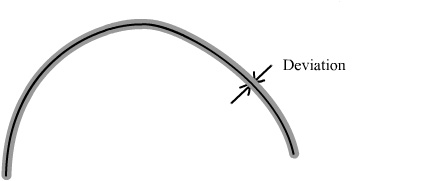For instance, shape has 2 curves (black). We want to replace them with single new curve (red). Most fit curve will be one that has minimum area or average length (depends on what optimization algorithm you have selected) between original curves and optimized one. In other words, Deviation value represents distance between original curves: the higher value you set, the more distant curves will be optimized into single one and, therefore, quality of resulting image will be worse.

• Curve to line replacement tolerance

All shapes have records of curves and lines which compose those shapes. Since size record of the line is smaller than size record of the curve then by replacing original curve with more or less identical line will save some space.

Curve to line replacement tolerance (rectify tolerance) sets limit which is a ratio between curve length and length of the line that connects to ends of the original curve.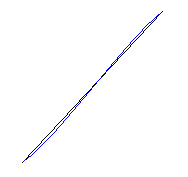By increasing tolerance value, more round shapes will be transformed to angular ones.

• Join Angle

This settings let you set limiting value of the tangent to the curves at the point of conjugation when replacing to curves by single one.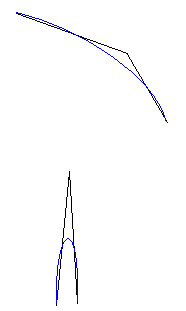The higher value of join angle you set the more sharp angles will be transformed into blunt ones and quality of the optimized shape can be essentially decreased.

• Corner optimization

Quite often angle in the shape is represented by 3 curves: two curves which represent edges of the angle, and a single one (shown blue at the picture), which joins other two.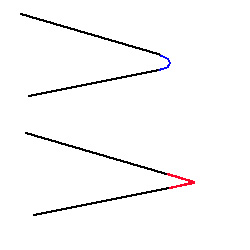Such angles can be optimized by removing small single curve and extending angle line to the point where it intersects (shown as red lines at the image above).